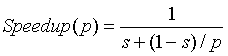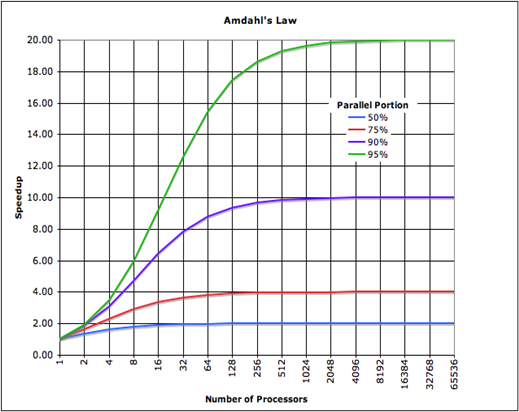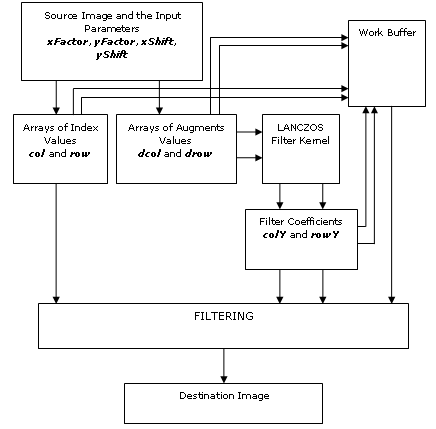# The Intel® AVX Realization of Lanczos interpolation in Intel® IPP 2D Resize Transform

ID 660353
Updated 8/31/2015
Version Latest
PublicBy

## Introduction

This paper presents the interpolation algorithm based on the Lanczos3 filter that is used in Intel® Integrated Performance Primitives (Intel® IPP). The use
of this algorithm gives 1.5 performance gains on the Intel® Advanced Vector Extensions (Intel® AVX) architecture comparing with the Intel SSE implementation.

Intel IPP implements the most popular algorithms from the simplest – nearest neighbor, bilinear – to the more sophisticated –supersampling
(the best image quality for reducing image size without any artifacts), different cubic filters, and so-called Lanczos3 filter. The last filter often allows
keeping the sharpness of lines with sufficient smoothness of the tonal transitions – much better than bicubic algorithms.

 Initial Image: 7x9 Pixels Nearest Neighbor Interpolation Mode Function parameters:                                xFactor = 83.05 yFactor = 83.05 xShift = -42.19 yShift = -124.24   ( ~83x enlargement)Linear Interpolation Mode Lanczos3 Interpolation ModeFigure 1. 2D Resize interpolation modeEquation 1. L(x) is the Lanczos Windowed Sinc (Integral sine) function

This algorithm uses 36 pixels of the source image for calculation the intensity of each pixel in the destination image.
The filter operation is rather expensive for each output pixel and perform 42 multiplications and 35 additions.
This algorithm is used in the functions ippiResizeSqrPixel when the parameter interpolation set to IPPI_INTER_LANCZOS.

This function resizes the source image ROI by xFactor in the x direction and yFactor in the y direction. The image size can be either reduced or increased
in each direction, depending on the values of xFactoryFactor. The result is resampled using the interpolation method specified by the interpolation
parameter and written to the destination image ROI. Pixel coordinates x' and y' in the resized image are obtained from the following equations:

x' = xFactor*x + xShift
y' = yFactor*y + yShift

Equation 2. 2D Resize transform (Forward)

Where and y denote the pixel coordinates in the source image.

The function requires the external buffer pBuffer, its size can be previously computed by calling the function ippiResizeGetBufSize.

## Flowchart of AlgorithmFigure 2. Pipeline

## Filtering

### Calculation of the Arrays of the Indexes

The first stage is the finding of the source pixels indexes needed for the interpolation task. It performs with the certain transforms – srcROI clipping and calculating its new coordinates after transforms with the specified parameters factors and shifts [Equation 2].

Then these coordinates is mapped back to source image. These transforms are detailed here.

x = (x' - xShift) / xFactor
y = (y' - yShift) / yFactor

Equation 3. 2D Resize Transform (inverse)

We find exact values of row indexes (int *row) and column indexes (int *col) and augment values (float *drow, float *dcol).

The augment value dcol is the distance along the X-axis between the integer coordinate (index) of the pixel in the source image and its coordinate (float) in the image obtained as a result
of the inverse transform. In addition, the augment value drow is the distance along the Y-axis between the integer coordinate (index) of the pixel in the source image and
its coordinate (float) in the image obtained as a result of the inverse transform [Equation 3].

#### Preparation of the Lanczos3 Filter

Before the interpolation the filter is applied [Equation 1].Figure 3. Three-lobed Lanczos window

The filter is implemented as a table tblLanczos3 (see The Filter Kernel).

The function ownLanczos3 using this table values is called two times:

1. For columns with parameters ownLanczos3(dcol, width, colY);
2. For rows with parameters ownLanczos3(drow, height, rowY);

Where dcol and drow are the augment values (see Calculation of the Arrays of the Indexes), width, and height are values of the processed image size, colY
and rowY are the float values after the Lanczos filter for horizontal and vertical interpolations.

This function is implemented in assembler, but we present here only its “c” analogue (see The Lanczos3 Filter Implementation).

#### Processing of the Possible Borders

It should be noted that in general case the resizing operation could require the border processing and the replication of the lacked border pixels. This is
performed by the special functions, and the vectoriazation and optimization cannot be applied. As the number of such pixels is too small, these functions
do not affect on the performance and are not considered here.

#### General Processing

The Lanczos interpolation proceeds by means of the ownResize32plLz function (see The General Processing Function), where the parameters
are the following:

float *startSrc \\is an input data
float *startDst \\is an output data
int srcStep \\is a step in source image
int dstStep \\is a step in destination image
int width, int height \\are proceed size values
int *row, int *col \\are values of the indexes
float *rowY, float *colY
\\are the interpolating coefficients by x- and y-directions
float *P0, float *P1, float *P2, float *P3, float *P4, float *P5
\\are the resultant values after interpolation by x-direction.

#### Interpolation by Rows (X-Direction)

The interpolation by rows is the most complicated and time-consuming phase of this processing.
It is realized by intrinsic function ownRowLanczos32pl (see AVX Implementation for Interpolation by X-Direction), where the parameters are following:

float *src \\is the data values of the sources image
int *col \\is the array of the index values
float *dY \\is the pre-calculated filter coefficients
\\(see Preparation of the Lanczos3 Filter)
float *P \\is the resultant values after interpolation
int width \\is the proceed length

The figure below shows how it can be done for eight pixels A, B, C, D, E, F, G and H using six YMM registers.P = A0*dY + A1*dY + A2*dY + A3*dY + A4*dY + A5*dY;
dY += 6;
P = B0*dY + B1*dY + B2*dY + B3*dY + B4*dY + B5*dY;
dY += 6;
P = C0*dY + C1*dY + C2*dY + C3*dY + C4*dY + C5*dY;
dY += 6;
P = D0*dY + D1*dY + D2*dY + D3*dY + D4*dY + D5*dY;
dY += 6;
P = E0*dY + E1*dY + E2*dY + E3*dY + E4*dY + E5*dY;
dY += 6;
P = F0*dY + F1*dY + F2*dY + F3*dY + F4*dY + F5*dY;
dY += 6;
P = G0*dY + G1*dY + G2*dY + G3*dY + G4*dY + G5*dY;
dY += 6;
P = H0*dY + H1*dY + H2*dY + H3*dY + H4*dY + H5*dY;
dY += 6;

This code is well suited for realization with 256-bit registers. The general feature is a usage of horizontal addition and new AVX shuffle instructions.

#### Interpolation by Columns (Y-Direction)

The interpolation by columns is realized by AVX intrinsics too – ownColLanczos32pl (see AVX Implementation for Interpolation by Y-Direction), where the parameters are following:

float *dst \\is the data values of the destination image
int width \\is the proceed length
float *dY \\is the pre-calculated filter coefficients by columns
\\(see Preparation of the Lanczos3 Filter)
float *P0, float *P1, float *P2, float *P3, float *P4, float *P5
\\are the values after interpolation by x-direction for needed six rows

## Summary

The presented AVX implementation of Lanczos interpolation for 2D resize transform has been estimated under simulator Coho and compared with
Intel SSE implementation.

The result is 1.5x faster.

Note These functions are implemented on intrinsics and require Intel® Compiler with AVX support (#include "gmmintrin.h")

## References

• George Wolberg, Digital Image Warping, IEEE Computer Society Press, Los Alamitos, California, 1994, page 142.
• Intel® Integrated Performance Primitives for Intel® Architecture, vol.2 – Image and Video Processing, Appendix B. Interpolation in Image Geometric Transform Functions, Lanczos Interpolation, B-7.
• Intel® Integrated Performance Primitives for Intel® Architecture, vol.2 – Image and Video Processing, Geometric Transform Functions, 12-12…12-14.

Yuri Tikhomirov is a Senior Software Engineer with the Software Solutions Group (Visual Computing Software Division, CIP, Intel IPP). He is focused on CPU-specific code development for Intel IPP libraries for all existing and future Intel Architectures. Yuri has been with Intel for five years.
His email is yuri.tikhomirov@intel.com.

## Appendix A: Example Code

### The Filter Kernel

1.00000000f,
0.99981719f, 0.99926907f, 0.99835593f, 0.99707854f, 0.99543774f, 0.99343479f,
0.99107116f, 0.98834860f, 0.98526901f, 0.98183489f, 0.97804850f, 0.97391278f,
0.96943063f, 0.96460551f, 0.95944083f, 0.95394045f, 0.94810826f, 0.94194865f,
0.93546599f, 0.92866510f, 0.92155081f, 0.91412848f, 0.90640318f, 0.89838088f,
0.89006704f, 0.88146782f, 0.87258941f, 0.86343807f, 0.85402042f, 0.84434319f,
0.83441335f, 0.82423782f, 0.81382394f, 0.80317909f, 0.79231060f, 0.78122634f,
0.76993400f, 0.75844145f, 0.74675679f, 0.73488796f, 0.72284341f, 0.71063137f,
0.69826019f, 0.68573844f, 0.67307454f, 0.66027725f, 0.64735508f, 0.63431686f,
0.62117124f, 0.60792708f, 0.59459317f, 0.58117831f, 0.56769133f, 0.55414099f,
0.54053622f, 0.52688581f, 0.51319844f, 0.49948296f, 0.48574796f, 0.47200218f,
0.45825425f, 0.44451272f, 0.43078604f, 0.41708267f, 0.40341082f, 0.38977870f,
0.37619469f, 0.36266673f, 0.34920263f, 0.33581027f, 0.32249743f, 0.30927145f,
0.29614016f, 0.28311053f, 0.27018979f, 0.25738502f, 0.24470302f, 0.23215052f,
0.21973385f, 0.20745964f, 0.19533394f, 0.18336278f, 0.17155206f, 0.15990727f,
0.14843382f, 0.13713717f, 0.12602237f, 0.11509412f, 0.10435715f, 0.09381592f,
0.08347469f, 0.07333750f, 0.06340817f, 0.05369032f, 0.04418736f, 0.03490248f,
0.02583865f, 0.01699864f, 0.00838497f, -0.00000000f, -0.00815418f, -0.01607572f,
-0.02376293f, -0.03121435f, -0.03842873f, -0.04540505f, -0.05214256f, -0.05864054f,
-0.06489867f, -0.07091672f, -0.07669481f, -0.08223311f, -0.08753207f, -0.09259231f,
-0.09741467f, -0.10200013f, -0.10634997f, -0.11046558f, -0.11434853f, -0.11800056f,
-0.12142362f, -0.12461984f, -0.12759149f, -0.13034099f, -0.13287102f, -0.13518427f,
-0.13728370f, -0.13917229f, -0.14085333f, -0.14233011f, -0.14360611f, -0.14468493f,
-0.14557026f, -0.14626595f, -0.14677592f, -0.14710422f, -0.14725500f, -0.14723253f,
-0.14704110f, -0.14668514f, -0.14616913f, -0.14549765f, -0.14467528f, -0.14370675f,
-0.14259681f, -0.14135024f, -0.13997187f, -0.13846658f, -0.13683930f, -0.13509491f,
-0.13323840f, -0.13127476f, -0.12920895f, -0.12704596f, -0.12479077f, -0.12244838f,
-0.12002371f, -0.11752179f, -0.11494751f, -0.11230581f, -0.10960151f, -0.10683950f,
-0.10402457f, -0.10116149f, -0.09825497f, -0.09530962f, -0.09233005f, -0.08932082f,
-0.08628634f, -0.08323110f, -0.08015937f, -0.07707538f, -0.07398339f, -0.07088737f,
-0.06779135f, -0.06469924f, -0.06161486f, -0.05854191f, -0.05548402f, -0.05244470f,
-0.04942736f, -0.04643530f, -0.04347174f, -0.04053976f, -0.03764234f, -0.03478234f,
-0.03196251f, -0.02918549f, -0.02645381f, -0.02376987f, -0.02113595f, -0.01855422f,
-0.01602676f, -0.01355540f, -0.01114205f, -0.00878838f, -0.00649594f, -0.00426619f,
-0.00210047f, 0.00000000f, 0.00203415f, 0.00400094f, 0.00589957f, 0.00772926f,
0.00948936f, 0.01117935f, 0.01279882f, 0.01434745f, 0.01582504f, 0.01723149f,
0.01856680f, 0.01983109f, 0.02102457f, 0.02214752f, 0.02320033f, 0.02418351f,
0.02509763f, 0.02594336f, 0.02672144f, 0.02743268f, 0.02807803f, 0.02865846f,
0.02917501f, 0.02962883f, 0.03002109f, 0.03035306f, 0.03062608f, 0.03084148f,
0.03100074f, 0.03110530f, 0.03115671f, 0.03115656f, 0.03110645f, 0.03100805f,
0.03086303f, 0.03067312f, 0.03044010f, 0.03016571f, 0.02985179f, 0.02950013f,
0.02911260f, 0.02869101f, 0.02823725f, 0.02775319f, 0.02724068f, 0.02670161f,
0.02613787f, 0.02555128f, 0.02494374f, 0.02431709f, 0.02367315f, 0.02301377f,
0.02234073f, 0.02165582f, 0.02096081f, 0.02025741f, 0.01954736f, 0.01883232f,
0.01811394f, 0.01739381f, 0.01667353f, 0.01595464f, 0.01523860f, 0.01452692f,
0.01382100f, 0.01312220f, 0.01243184f, 0.01175121f, 0.01108153f, 0.01042398f,
0.00977970f, 0.00914975f, 0.00853517f, 0.00793692f, 0.00735592f, 0.00679305f,
0.00624910f, 0.00572484f, 0.00522095f, 0.00473809f, 0.00427684f, 0.00383773f,
0.00342123f, 0.00302778f, 0.00265773f, 0.00231140f, 0.00198902f, 0.00169082f,
0.00141693f, 0.00116746f, 0.00094244f, 0.00074186f, 0.00056567f, 0.00041376f,
0.00028596f, 0.00018208f, 0.00010186f, 0.00004501f, 0.00001118f, 0.00000000f,
0.00000000f
};

### The Lanczos3 Filter Implementation

void ownLanczos3 (float *dcr, int length, float *dY)
{
int i, n, ind;
float dL, indf, norm;
for (i = 0; i < length; i ++) {
dL = -2 - dcr[i];
norm = 0.f;
for (n = 0; n < 6; n ++) {
if ((dL > -3.0) && (dL < 3.0)) {
indf = (float)(fabs(dL) * 100);
ind = (int)indf;
dY[n] = tblLanczos3[ind] + (tblLanczos3[ind+1] - tblLanczos3[ind]) * (indf - ind);
} else { dY[n] = 0; }
norm += dY[n];
dL ++;
}
for (n = 0; n < 6; n ++) dY[n] /= norm;
dY += 6;
}
}

### The General Processing Function

void ownResize32plLz (
float *startSrc, float *startDst, int srcStep, int dstStep,
int width, int height, int *row, int *col, float *rowY, float *colY,
float *P0, float *P1, float *P2, float *P3, float *P4, float *P5)
{
float *Pt;
int j, nRow, tRow;
int srcStep2, srcStep3, srcStep4, srcStep5, srcStep6;

srcStep2 = srcStep + srcStep;
srcStep3 = srcStep2 + srcStep;
srcStep4 = srcStep3 + srcStep;
srcStep5 = srcStep4 + srcStep;
srcStep6 = srcStep5 + srcStep;
ownRowLanczos32pl(startSrc + row - srcStep2, col, colY, P1, width);
ownRowLanczos32pl(startSrc + row - srcStep , col, colY, P2, width);
ownRowLanczos32pl(startSrc + row , col, colY, P3, width);
ownRowLanczos32pl(startSrc + row + srcStep , col, colY, P4, width);
ownRowLanczos32pl(startSrc + row + srcStep2, col, colY, P5, width);
nRow = (srcStep > 0) ? (row - 1) : (row + 1);
for (j = 0; j < height; j ++) {
tRow = row[j];
if (srcStep > 0) { /* positive step */
if (tRow > nRow) {
Pt = P0; P0 = P1; P1 = P2; P2 = P3; P3 = P4; P4 = P5; P5 = Pt;
ownRowLanczos32pl(startSrc + tRow + srcStep3, col, colY, P5, width);
if (tRow >= nRow + srcStep2) {
Pt = P0; P0 = P1; P1 = P2; P2 = P3; P3 = P4; P4 = Pt;
ownRowLanczos32pl(startSrc + tRow + srcStep2, col, colY, P4, width);
}
if (tRow >= nRow + srcStep3) {
Pt = P0; P0 = P1; P1 = P2; P2 = P3; P3 = Pt;
ownRowLanczos32pl(startSrc + tRow + srcStep, col, colY, P3, width);
}
if (tRow >= nRow + srcStep4) {
Pt = P0; P0 = P1; P1 = P2; P2 = Pt;
ownRowLanczos32pl(startSrc + tRow, col, colY, P2, width);
}
if (tRow >= nRow + srcStep5) {
Pt = P0; P0 = P1; P1 = Pt;
ownRowLanczos32pl(startSrc + tRow - srcStep, col, colY, P1, width);
}
if (tRow >= nRow + srcStep6) {
ownRowLanczos32pl(startSrc + tRow - srcStep2, col, colY, P0, width);
}
nRow = tRow;
}
}
else { /* negative step */
if (tRow < nRow) {
Pt = P0; P0 = P1; P1 = P2; P2 = P3; P3 = P4; P4 = P5; P5 = Pt;
ownRowLanczos32pl(startSrc + tRow + srcStep3, col, colY, P5, width);
if (tRow <= nRow + srcStep2) {
Pt = P0; P0 = P1; P1 = P2; P2 = P3; P3 = P4; P4 = Pt;
ownRowLanczos32pl(startSrc + tRow + srcStep2, col, colY, P4, width);
}
if (tRow <= nRow + srcStep3) {
Pt = P0; P0 = P1; P1 = P2; P2 = P3; P3 = Pt;
ownRowLanczos32pl(startSrc + tRow + srcStep, col, colY, P3, width);
}
if (tRow <= nRow + srcStep4) {
Pt = P0; P0 = P1; P1 = P2; P2 = Pt;
ownRowLanczos32pl(startSrc + tRow, col, colY, P2, width);
}
if (tRow <= nRow + srcStep5) {
Pt = P0; P0 = P1; P1 = Pt;
ownRowLanczos32pl(startSrc + tRow - srcStep, col, colY, P1, width);
}
if (tRow <= nRow + srcStep6) {
ownRowLanczos32pl(startSrc + tRow - srcStep2, col, colY, P0, width);
}
nRow = tRow;
}
}

/* interpolation by columns */
ownColLanczos32pl(startDst, width, rowY, P0, P1, P2, P3, P4, P5);
rowY += 6;
startDst += dstStep;
}
}

### AVX Implementation for Interpolation by X-Direction

static __declspec (align(16)) int Yperm_msk = {0, 1, 6, 7, 12, 13, 10, 11};

void ownRowLanczos32pl (
float *src, int *col, float *dY, float *P, int width)
{
int wid8, wid4, i;
__declspec (align(16)) __m128 Xt0, Xt1, Xt2, Xt3, Xt4, Xt5;
__declspec (align(16)) __m256 Yt0, Yt1, Yt2, Yt3, Yt4, Yt5;

wid8 = (width >> 3) << 3;
wid4 = (width - wid8) & 4;
for (i = 0; i < wid8; i += 8) {

/* B1 B0 A5 A4 A3 A2 A1 A0 */
Yt0 = _mm256_insertf128_ps(_mm256_cast_ps128_ps256(Xt0), Xt1, 1);
/* C3 C2 C1 C0 B5 B4 B3 B2 */
Yt1 = _mm256_insertf128_ps(_mm256_cast_ps128_ps256(Xt2), Xt3, 1);
/* D5 D4 D3 D2 D1 D0 C5 C4 */
Yt2 = _mm256_insertf128_ps(_mm256_cast_ps128_ps256(Xt4), Xt5, 1);

/* F1 F0 E5 E4 E3 E2 E1 E0 */
Yt3 = _mm256_insertf128_ps(_mm256_cast_ps128_ps256(Xt0), Xt1, 1);
/* G3 G2 G1 G0 F5 F4 F3 F2 */
Yt4 = _mm256_insertf128_ps(_mm256_cast_ps128_ps256(Xt2), Xt3, 1);
/* H5 H4 H3 H2 H1 H0 G5 G4 */
Yt5 = _mm256_insertf128_ps(_mm256_cast_ps128_ps256(Xt4), Xt5, 1);
Yt0 = _mm256_mul_ps(Yt0, *(__m256*)dY);
Yt1 = _mm256_mul_ps(Yt1, *(__m256*)(dY+8));
Yt2 = _mm256_mul_ps(Yt2, *(__m256*)(dY+16));
Yt3 = _mm256_mul_ps(Yt3, *(__m256*)(dY+24));
Yt4 = _mm256_mul_ps(Yt4, *(__m256*)(dY+32));
Yt5 = _mm256_mul_ps(Yt5, *(__m256*)(dY+40));

/* C2+C3 C0+C1 B0+B1 A4+A5 | B4+B5 B2+B3 A2+A3 A0+A1 */
/* G2+G3 G0+G1 F0+F1 E4+E5 | F4+F5 F2+F3 E2+E3 E0+E1 */
/* D4+D5 D2+D3 C2+C3 C0+C1 | D0+D1 C4+C5 B4+B5 B2+B3 */
/* H4+H5 H2+H3 G2+G3 G0+G1 | H0+H1 G4+G5 F4+F5 F2+F3 */

/* F4+F5 F2+F3 E2+E3 E0+E1 | B4+B5 B2+B3 A2+A3 A0+A1 */
Yt1 = _mm256_permute2f128_ps(Yt0, Yt3, 0x20);
/* H4+H5 H2+H3 G2+G3 G0+G1 | D4+D5 D2+D3 C2+C3 C0+C1 */
Yt4 = _mm256_permute2f128_ps(Yt2, Yt5, 0x31);
/* H0+H1 G4+G5 xxxxx xxxxx | xxxxx xxxxx B0+B1 A4+A5 */
Yt0 = _mm256_permute2f128_ps(Yt0, Yt5, 0x21);
/* xxxxx xxxxx F0+F1 E4+E5 | D0+D1 C4+C5 xxxxx xxxxx */
Yt2 = _mm256_permute2f128_ps(Yt2, Yt3, 0x30);
/* H2345 G0123 F2345 E0123 | D2345 C0123 B2345 A0123 */
Yt0 = _mm256_permute2_ps(Yt0, Yt2, *(__m256i*)Yperm_msk, 0);

dY += 48; P += 8;
}

if (wid4) {
Yt0 = _mm256_insertf128_ps(_mm256_cast_ps128_ps256(Xt0), Xt1, 1);
Yt1 = _mm256_insertf128_ps(_mm256_cast_ps128_ps256(Xt2), Xt3, 1);
Yt2 = _mm256_insertf128_ps(_mm256_cast_ps128_ps256(Xt4), Xt5, 1);
Yt0 = _mm256_mul_ps(Yt0, *(__m256*)dY);
Yt1 = _mm256_mul_ps(Yt1, *(__m256*)(dY+8));
Yt2 = _mm256_mul_ps(Yt2, *(__m256*)(dY+16));
Xt1 = _mm256_extractf128_ps(Yt0, 1);
Xt0 = _mm256_extractf128_ps(Yt2, 1);
Xt1 = _mm_shuffle_ps(Xt1, _mm256_cast_ps256_ps128(Yt2), 0xe4);
dY += 24; P += 4; i += 4;
}
for (; i < width; i ++) {
Xt0 = _mm_mul_ps(Xt0, *(__m128*)dY);
Xt1 = _mm_mul_ps(Xt1, Xt2);
Xt0 = _mm_add_ss(Xt0, _mm_shuffle_ps(Xt0, Xt0, 1));
_mm_store_ss(P, Xt0);
dY += 6; P ++;
}
}

### AVX Implementation for Interpolation by Y-Direction

#define CALC_DST8
Yt0 = _mm256_mul_ps(Yt0, Yy0);
Yt1 = _mm256_mul_ps(Yt1, Yy1);
Yt2 = _mm256_mul_ps(Yt2, Yy2);
Yt3 = _mm256_mul_ps(Yt3, Yy3);
Yt4 = _mm256_mul_ps(Yt4, Yy4);
Yt5 = _mm256_mul_ps(Yt5, Yy5);

#define CALC_DST4
Xt0 = _mm_mul_ps(Xt0, Xy0);
Xt1 = _mm_mul_ps(Xt1, Xy1);
Xt2 = _mm_mul_ps(Xt2, Xy2);
Xt3 = _mm_mul_ps(Xt3, Xy3);
Xt4 = _mm_mul_ps(Xt4, Xy4);
Xt5 = _mm_mul_ps(Xt5, Xy5);

#define CALC_DST1
Xt0 = _mm_mul_ss(Xt0, Xy0);
Xt1 = _mm_mul_ss(Xt1, Xy1);
Xt2 = _mm_mul_ss(Xt2, Xy2);
Xt3 = _mm_mul_ss(Xt3, Xy3);
Xt4 = _mm_mul_ss(Xt4, Xy4);
Xt5 = _mm_mul_ss(Xt5, Xy5);

__INLINE
__int64 IINT_PTR (const void* ptr)
{
union {
void* Ptr;
__int64 Int;
} dd;
dd.Ptr = (void*)ptr;
return dd.Int;
}

void ownColLanczos32pl (float *dst, int width, float *dY,
float *P0, float *P1, float *P2, float *P3, float *P4, float *P5)
{
int wid8, wid4, i;
__declspec (align(16)) __m128 Xt0, Xt1, Xt2, Xt3, Xt4, Xt5, Xy0, Xy1, Xy2, Xy3, Xy4, Xy5;
__declspec (align(16)) __m256 Yt0, Yt1, Yt2, Yt3, Yt4, Yt5, Yy0, Yy1, Yy2, Yy3, Yy4, Yy5;

wid8 = (width >> 3) << 3;
wid4 = (width - wid8) & 4;

Yy0 = _mm256_insertf128_ps(_mm256_cast_ps128_ps256(Xy0), Xy0, 1);
Yy1 = _mm256_insertf128_ps(_mm256_cast_ps128_ps256(Xy1), Xy1, 1);
Yy2 = _mm256_insertf128_ps(_mm256_cast_ps128_ps256(Xy2), Xy2, 1);
Yy3 = _mm256_insertf128_ps(_mm256_cast_ps128_ps256(Xy3), Xy3, 1);
Yy4 = _mm256_insertf128_ps(_mm256_cast_ps128_ps256(Xy4), Xy4, 1);
Yy5 = _mm256_insertf128_ps(_mm256_cast_ps128_ps256(Xy5), Xy5, 1);

if (!(IINT_PTR(dst) & 31)) { /* dst pointer aligned on 32 */
for (i = 0; i < wid8; i += 8) {
CALC_DST8
_mm256_store_ps(dst, Yt0);
P0 += 8; P1 += 8; P2 += 8; P3 += 8; P4 += 8; P5 += 8; dst += 8;
}
if (wid4) {
CALC_DST4
_mm_store_ps(dst, Xt0);
P0 += 4; P1 += 4; P2 += 4; P3 += 4; P4 += 4; P5 += 4; dst += 4; i += 4;
}
}
else {
for (i = 0; i < wid8; i += 8) {
CALC_DST8
_mm256_storeu_ps(dst, Yt0);
P0 += 8; P1 += 8; P2 += 8; P3 += 8; P4 += 8; P5 += 8; dst += 8;
}
if (wid4) {
CALC_DST4
_mm_storeu_ps(dst, Xt0);
P0 += 4; P1 += 4; P2 += 4; P3 += 4; P4 += 4; P5 += 4; dst += 4; i += 4;
}
}
for (; i < width; i ++) {
CALC_DST1
_mm_store_ss(dst, Xt0);
P0 ++; P1 ++; P2 ++; P3 ++; P4 ++; P5 ++; dst ++;
}
}